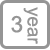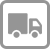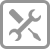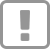• 产品详情

--厨房空气净化专家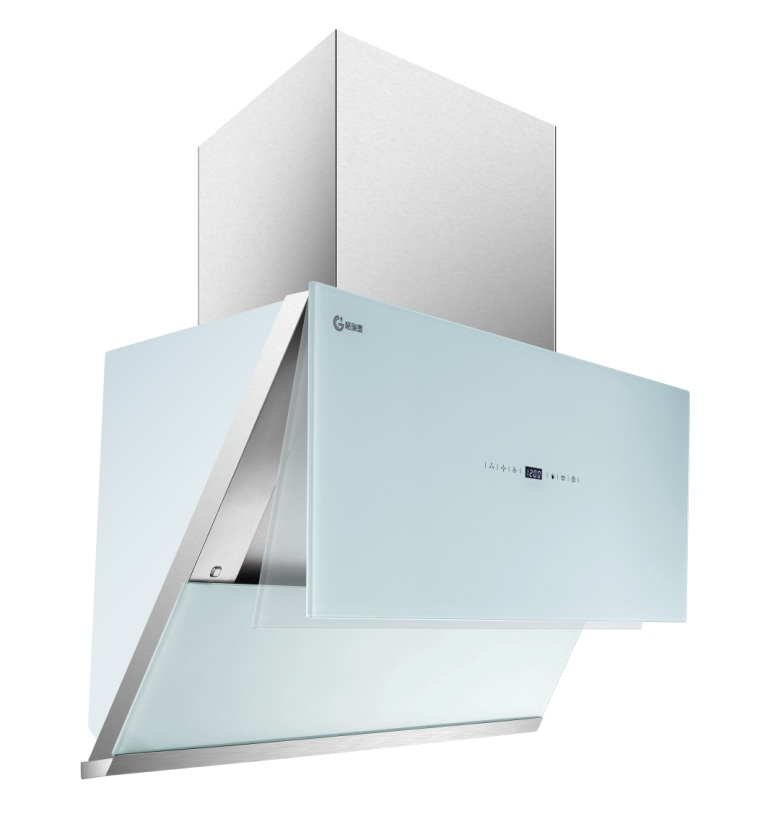^^^^^^^^^^^^^^^^^^^^^^^^^^^^^^^^^^^^^^^^^^^^^^^^^^^^^^^^^^^^^^^^^^^^^^^^^^^^^^^^^^^

3200cm2的“巨幕拢烟屏”，能带来深达16000cm³的有效拢烟区，形成一个超大倒锥漏斗负压区，“近距劲吸”、“深腔拢烟”与“屏随烟动”三大核心技术智能配合，对油烟进行智能操控，实现油烟全吸走。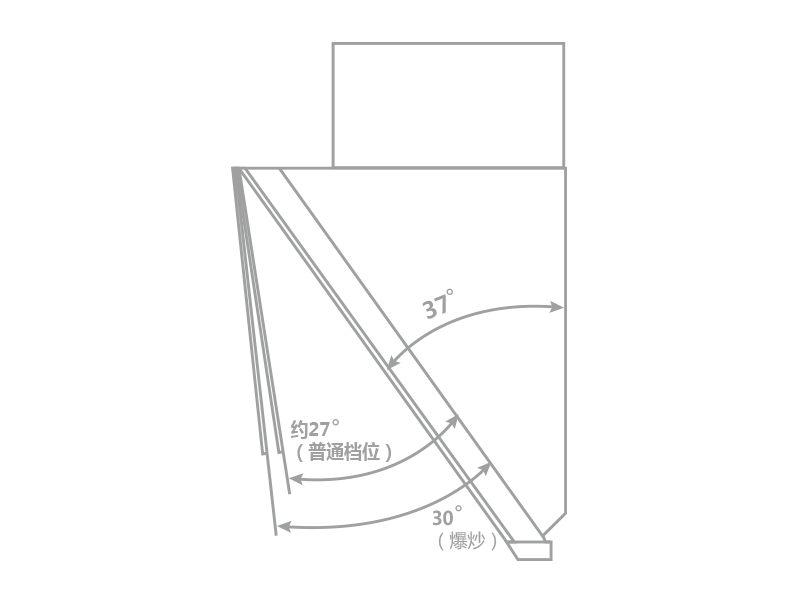^^^^^^^^^^^^^^^^^^^^^^^^^^^^^^^^^^^^^^^^^^^^^^^^^^^^^^^^^^^^^^^^^^^^^^^^^^^^^^^^^^^

>

Professional security care for a better life

【享受服务】